Test: Frequency Modulation

# Test: Frequency Modulation - Electronics and Communication Engineering (ECE)

Test Description

## 10 Questions MCQ Test GATE ECE (Electronics) 2023 Mock Test Series - Test: Frequency Modulation

Test: Frequency Modulation for Electronics and Communication Engineering (ECE) 2023 is part of GATE ECE (Electronics) 2023 Mock Test Series preparation. The Test: Frequency Modulation questions and answers have been prepared according to the Electronics and Communication Engineering (ECE) exam syllabus.The Test: Frequency Modulation MCQs are made for Electronics and Communication Engineering (ECE) 2023 Exam. Find important definitions, questions, notes, meanings, examples, exercises, MCQs and online tests for Test: Frequency Modulation below.
Solutions of Test: Frequency Modulation questions in English are available as part of our GATE ECE (Electronics) 2023 Mock Test Series for Electronics and Communication Engineering (ECE) & Test: Frequency Modulation solutions in Hindi for GATE ECE (Electronics) 2023 Mock Test Series course. Download more important topics, notes, lectures and mock test series for Electronics and Communication Engineering (ECE) Exam by signing up for free. Attempt Test: Frequency Modulation | 10 questions in 30 minutes | Mock test for Electronics and Communication Engineering (ECE) preparation | Free important questions MCQ to study GATE ECE (Electronics) 2023 Mock Test Series for Electronics and Communication Engineering (ECE) Exam | Download free PDF with solutions
 1 Crore+ students have signed up on EduRev. Have you?
Test: Frequency Modulation - Question 1

### An FM signal is given as;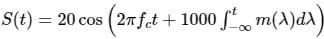Where m(t) is shown in the figure given below.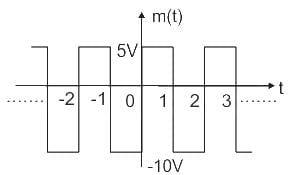It is given that carrier frequency (fc) is 1000kHz. Then calculate the value of instantaneous frequency (fi) at t = 1.5 sec and the peak frequency deviation (δf) of the above FM signal.

Detailed Solution for Test: Frequency Modulation - Question 1

Concept:
Standard expression of FM signal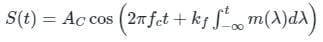Instantaneous frequency (fi in Hz)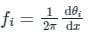Where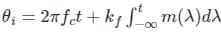From the standard expression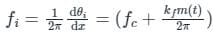Peak frequency deviation: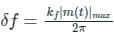Calculation:
Given;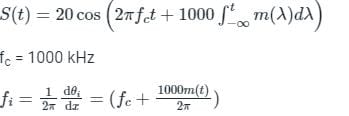at, t = 1.5 sec ; m(t) = -10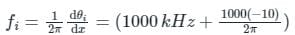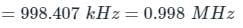Peak frequency deviation:
|m(t)|max = 10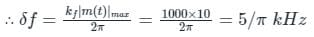Test: Frequency Modulation - Question 2

### A phase modulated wave can be generated from frequency modulator by connecting a ___________ before the modulator and a frequency modulated wave can be generated from phase modulator by connecting a __________ before the modulator.

Detailed Solution for Test: Frequency Modulation - Question 2

The output of FM modulator is of form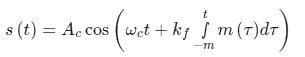After passing the modulating signal through the differentiator we give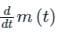as the input to the frequency modulator, i.e.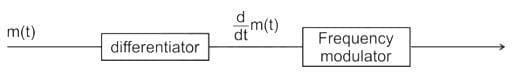The expression of the output signal will be: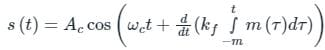The differentiation of the integral will simply give the original signal.
We get a phase modulated signal which is of the form: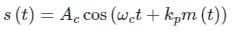Test: Frequency Modulation - Question 3

### Frequency modulated signal with single tone modulation has a frequency deviation of 20 kHz and bandwidth of 60 kHz. The frequency of the modulating signal will be -

Detailed Solution for Test: Frequency Modulation - Question 3

Concept:
As per Carson’s rule, the bandwidth of a frequency modulated signal is defined as:
B.W. = 2 (Δf + fm)
Δ f = Frequency deviation of the modulated wave
fm = frequency of the modulating signal

Calculation:
Given B.W. = 60 kHz and Δ f = 20 kHz
60k = 2 (20k + fm)
30k = 20k + fm
fm = 10 kHz

Test: Frequency Modulation - Question 4

Which is true in a frequency modulated system ?

Detailed Solution for Test: Frequency Modulation - Question 4

Frequency Modulation:

• Frequency Modulation is a modulation in which the frequency of the carrier wave is altered according to the instantaneous amplitude of the modulating signal, keeping phase and amplitude constant.
• So, the variation in carrier amplitude and carrier phase does not affect the signal in the receiving end.
• Line of sight (LoS) is a type of propagation that can transmit and receive data only where transmit and receive stations are in view of each other without any sort of an obstacle between them. Eg: FM radio, microwave, and satellite transmission.
• Frequency Modulation works on the Line of sight propagation.

Types of FM detection:

• Slope detection
• Phase-locked loop detection
• Foster Seeley detection
• Ratio detector
Test: Frequency Modulation - Question 5

Foster Seeley discriminator uses a

Detailed Solution for Test: Frequency Modulation - Question 5

Foster Seeley discriminator:

• Discriminator circuits can generate electrical output directly proportional to the frequency deviation from the unmodulated RF carrier frequency.
• The simplest circuit could be a balanced slope detector.
• It makes use of two resonant circuits; one off-tuned to one side of unmodulated RF carrier frequency and the other off-tuned to the other side of it.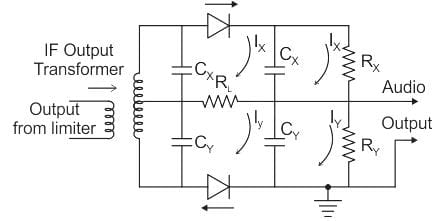• Another class of FM detectors known as Quadrature detectors uses a combination of two Quadrature signals.
• The Most commonly used FM detectors namely the foster-Seeley FM discriminator and the ratio detector operates on the principle of quadrature.

Foster Seeley discriminator is used in the demodulation of FM signal and it uses a double-tuned circuit with primary and secondary tuned to the same frequency.

Test: Frequency Modulation - Question 6

In a frequency modulation system, maximum frequency deviation allowed is 1000 and modulating frequency is 1 kHz. Determine modulation index β.

Detailed Solution for Test: Frequency Modulation - Question 6

Concept:
In FM (Frequency Modulation), the modulation index is defined as the ratio of frequency deviation to the modulating frequency.
Mathematically, this is defined as: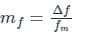mf = Modulation index
Δf = Frequency deviation
fm = Modulating frequency

Calculation:
Given Δf = 1000 Hz = 1 kHz
fm = 1 kHz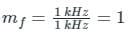Test: Frequency Modulation - Question 7

The four basic elements in a PLL are loop filter, loop amplifier, VCO and

Detailed Solution for Test: Frequency Modulation - Question 7
• A phase-locked loop is a control system that generates an output signal whose phase is related to the phase of an input signal.
• The circuit can track an input frequency or it can generate a frequency that is a multiple of the input frequency.
• The basic elements of a PLL circuit are phase comparator/detector, a loop filter, voltage controlled oscillator (VCO).

The figure shows a schematic of a phase-locked loop: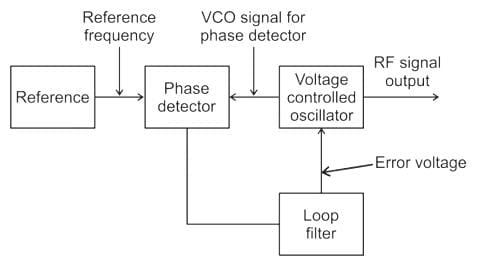Test: Frequency Modulation - Question 8

Determine the bandwidth required for an FM signal having frequency 2 kHz and maximum deviation 10 kHz.

Detailed Solution for Test: Frequency Modulation - Question 8

Concept:
According to Carson rule signal, BW is given as:
B.W = (β + 1) 2fm
∵ β = Δf/fm
B.W. = 2[Δf + fm]
If multiple frequencies are available in modulating signal then,
B.W. = 2(β + 1) fmax

Calculation:
Given:
Δf = 10 kHz
fm = 2 kHz
B.W. = 2[Δf + fm]
B.W. = 2(10 + 2)
BW = 24 kHz
Hence option (3) is the correct answer.

Test: Frequency Modulation - Question 9

In frequency modulation

Detailed Solution for Test: Frequency Modulation - Question 9

A wave has 3 parameters Amplitude, Phase, and Frequency. Thus there are 3 types of modulation techniques.

• Amplitude Modulation: The amplitude of the carrier is varied according to the message signal.
• Frequency Modulation: The frequency of the carrier is varied according to the message signal.
• Phase Modulation: The Phase of the carrier is varied according to the message signal.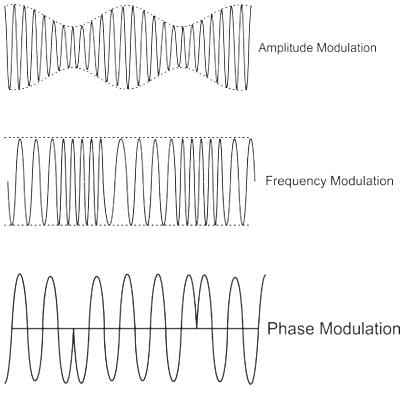Test: Frequency Modulation - Question 10

Consider the FM signal xc(t) = 10 cos(2π × 108t + 0.5 sin(104 πt)). The bandwidth of xc(t) is approximately

Detailed Solution for Test: Frequency Modulation - Question 10

The Bandwidth of an FM signal is approximated by Carlson’s rule and is given by
B.W. = 2(Δf + fm).
Where Δf = maximum frequency deviation from the carrier frequency and fm = message signal frequency.

Calculation:
Given, SFM(t) = 10 cos (2π × 108 t + 0.5 sin (104πt))
The instantaneous frequency is given by: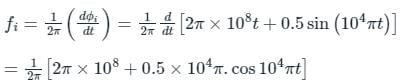⇒ Maximum instantaneous frequency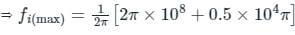Maximum frequency deviation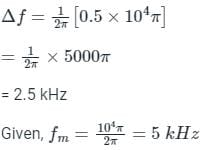So, Approximate bandwidth according to Carson’s rule
⇒ 2(Δf + fm) = 2(2.5 + 5) = 2 (7.5 K) = 15 K

## GATE ECE (Electronics) 2023 Mock Test Series

21 docs|263 tests
Information about Test: Frequency Modulation Page
In this test you can find the Exam questions for Test: Frequency Modulation solved & explained in the simplest way possible. Besides giving Questions and answers for Test: Frequency Modulation, EduRev gives you an ample number of Online tests for practice

## GATE ECE (Electronics) 2023 Mock Test Series

21 docs|263 tests(Scan QR code)